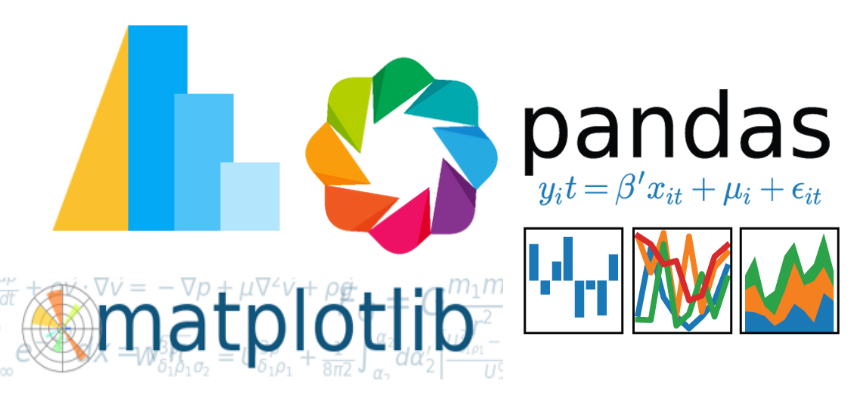There are many different plotting libraries in the Python ecosystem. Matplotlib is widley used, but there are other alternatives. After I watched a webinar from the Anaconda folks about all the possible plotting options Taming the Python Visualization Jungle and heard a podcast.__init__ episode about Data Science For Academic Research with Jake Vanderplas, I wanted to try using a few new Python plotting libraries and compare them to Matplotlib.

In this post, we're going to plot the same stress-strain curve using four different Python plotting libraries.

The four plotting libraries are:

The data we are going to plot is from a mechanical test frame. Mechanical test frames are used to test the mechanical properties of materials. The test frame pulls a sample apart. As the frame extends, the amount of force and the amount of extension are measured and recorded. The data from the mechanical test frame was saved in the form of a .csv file. We'll plot a stress-strain curve with the four libraries using this data.

Before we can build any plots, we need to make sure the plotting libraries are installed in our current working Python environment.

### Installation¶

All four of the plotting libraries can be installed at the Anaconda Prompt. Let's build a virtual environment too, and then install the plotting libraries into the new virtual environment. It's easy to use all four libraries in a Jupyter notebook, so we'll install Jupyter as well. The command jupyter notebook will start the Jupyter notebook application.

> conda create -n plotting python=3.7
> conda activate plotting
(plotting)> conda install pandas matplotlib bokeh holoviews
(plotting)> conda install -c conda-forge altair vega_datasets notebook vega
(plotting)> conda install jupyter
(plotting)> jupyter notebook


### 1. Pandas¶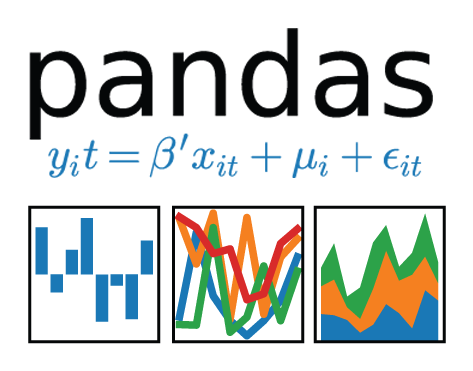First up is Pandas. Pandas is really a library for dealing with tabular data, not plotting. But if we use Pandas to import data, clean data, and rearrange data, we might as well try plotting with it too. Plotting in Pandas is quick and easy.

In :
import pandas as pd
%matplotlib inline
print(f'Pandas version: {pd.__version__}')

Pandas version: 0.23.4


The same data will be used to build each plot. It is pretty easy to load the raw data into a Pandas dataframe. The data is in two columns, the first column is the strain, which will be the x-values in each plot. The second column is stress, which will be the y-values in each plot.

In :
df = pd.read_csv('stress_strain_data.csv', sep=',', header=None, skiprows=0)
df.columns = ['strain', 'stress']

Out:
strain stress
0 0.000000 0.000
1 0.000605 43.821
2 0.001211 74.356
3 0.001816 104.930
4 0.002421 137.510

Building a simple plot with Pandas requires the df.plot() method call. The keyword arguments (x='strain', y='stress') are passed into the method. This puts strain on the x-axis and stress on the y-axis. df.plot() produces a pretty basic plot, but it sure is quick. Besides the import lines, that's two lines of code to build a plot in Python.

In :
df.plot(x='strain', y='stress')

Out:
<matplotlib.axes._subplots.AxesSubplot at 0x1e1c9dce9e8>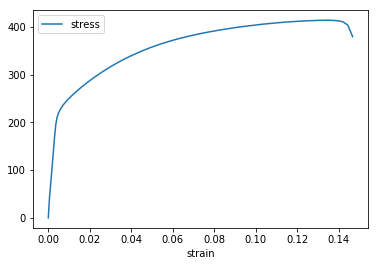### 2. Matplotlib¶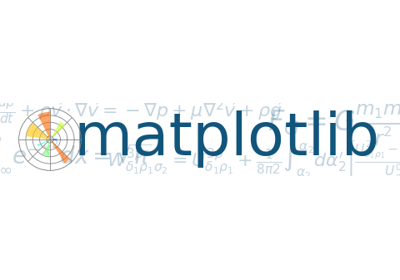Matplotlib is the plotting library I'm the most familiar with. Matplotlib is also one of the oldest and most used Python plotting libraries. Before building the plot, pulling the strain and stress columns out of dataframe allows us to set the columns as the x-values and y-values. Compared to Pandas, Matplotlib allows a lot more customization.

In :
import matplotlib.pyplot as plt
import pandas as pd
%matplotlib inline

df.columns = ['strain', 'stress']

strain = df['strain']
stress = df['stress']
plt.plot(strain,stress)
plt.xlabel('Strain (mm/mm)')
plt.ylabel('Stress (MPa)')
plt.title('matplotlib')
plt.show()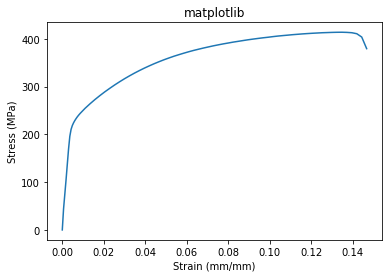### 3. Altair¶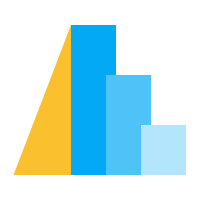Altair is a new library for me. It was created by the awesome Jake Vanderplas as a more portable plotting option compared to Matplotlib. The Altair documentation recommends installing Altair with either pip or conda. I used the conda command to install Altair from the conda-forge channel. Altair is not included in the Anaconda distribution of Python. So if you are using Anaconda, Altair must be installed seperatly. The Altair documentation states that vega_datasets and vega need to be installed (as well as Altair) in order to use Altair in a Jupyter notebook. To render altair plots in a Jupyter notebook the line alt.renderers.enable('notebook') needs be included below the imports.

> conda install -c conda-forge altair vega_datasets notebook vega

In :
import altair as alt
alt.renderers.enable('notebook')
import pandas as pd
print("Altair Version: ", alt.__version__)

Altair Version:  2.2.2

In :
df = pd.read_csv('stress_strain_data.csv', sep=',', header=None, skiprows=0)
df.columns = ['strain', 'stress']

alt.Chart(df).mark_line().encode(
x='strain',
y='stress'
)

Out: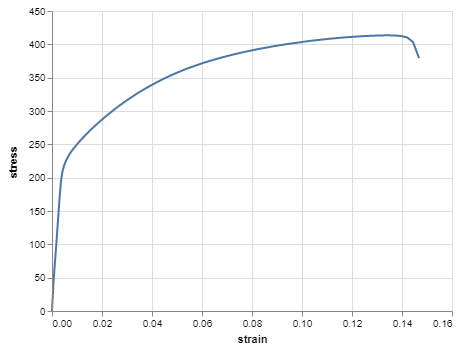### 4. Bokeh (with Holoviews)¶The final plotting library is Bokeh. Bokeh plots are designed for web viewing. Bokeh plots include a set of tools that allows zoom, pan, saving, and reloading.

I find the Bokeh API pretty complex. There are so many options, it can be difficult to get a simple plot like our stress-strain curve up and running. Luckily, there is a wrapper for Bokeh called Holoviews that makes using Bokeh easier for simple plots (like our stress-strain curve). To install Bokeh and Holoviews, I used conda :

> conda install bokeh
> conda install holoviews


If using a Jupyter notebook, calling the hv.Curve() method will produce a plot. I am saving the plot .html and re-displaying it using Jupyter's HTML() function. This just allows me to put the plot on my blog.

In :
import pandas as pd
import holoviews as hv
hv.extension('bokeh')
print(f'Holoviews verion: {hv.__version__}')